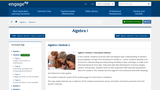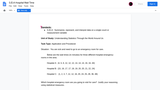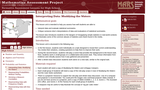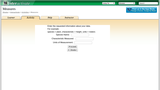# Search Results (15)

View
Selected filters:
• WI.Math.Content.HSS-ID.A.3Conditional Remix & Share Permitted
CC BY-NC-SA
Rating

This task examines, from a mathematical and statistical point of view, how ...

This task examines, from a mathematical and statistical point of view, how scientists measure the age of organic materials by measuring the ratio of Carbon 14 to Carbon 12. The focus here is on the statistical nature of such dating. This task addresses a very important issue about precision in reporting and understanding statements in a realistic scientific context.

Subject:
Mathematics
Material Type:
Activity/Lab
Provider:
Illustrative Mathematics
Provider Set:
Illustrative Mathematics
Author:
Illustrative Mathematics
09/13/2012Conditional Remix & Share Permitted
CC BY-NC-SA
Rating

In this module, students reconnect with and deepen their understanding of statistics ...

In this module, students reconnect with and deepen their understanding of statistics and probability concepts first introduced in Grades 6, 7, and 8. Students develop a set of tools for understanding and interpreting variability in data, and begin to make more informed decisions from data. They work with data distributions of various shapes, centers, and spreads. Students build on their experience with bivariate quantitative data from Grade 8. This module sets the stage for more extensive work with sampling and inference in later grades.

Subject:
Statistics and Probability
Material Type:
Module
Provider:
New York State Education Department
Provider Set:
EngageNY
08/01/2013Conditional Remix & Share Permitted
CC BY-NC-SA
Rating

This Desmos activity provides an interactive opportunity for students to work with ...

This Desmos activity provides an interactive opportunity for students to work with data as represented  between data and the box plot that results from it. Students walk through an investigation on interpreting center, spread, and the impact of outliers on various box plots. Students will also use their inference skills to compare two box plots. The teacher has the ability to pace students on slides, pause them, and promote whole class discussion based upon individual student responses. The teacher can also anonymize students names to provide for more risk taking in their responses.Students may be reminded of their prior knowledge of a box plot from middle school before starting this lesson.This original lesson was created by Bob Lochel and modified by Emily O'Brien.

Subject:
Information and Technology Literacy
Mathematics
Material Type:
Lesson Plan
Author:
Emily O'Brien
03/11/2019Conditional Remix & Share Permitted
CC BY-NC-SA
Rating

This task requires students to use the normal distribution as a model ...

This task requires students to use the normal distribution as a model for a data distribution. Students must use given means and standard deviations to approximate population percentages. There are several ways (tables, graphing calculators, or statistical software) that students might calculate the required normal percentages. Depending on the method used, answers might vary somewhat from those shown in the solution.

Subject:
Mathematics
Material Type:
Activity/Lab
Provider:
Illustrative Mathematics
Provider Set:
Illustrative Mathematics
Author:
Illustrative Mathematics
01/02/2013Conditional Remix & Share Permitted
CC BY-NC-SA
Rating

This problem could be used as an introductory lesson to introduce group ...

This problem could be used as an introductory lesson to introduce group comparisons and to engage students in a question they may find amusing and interesting. More generally, the idea of the lesson could be used as a template for a project where students develop a questionnaire, sample students at their school and report on their findings.

Subject:
Mathematics
Material Type:
Activity/Lab
Provider:
Illustrative Mathematics
Provider Set:
Illustrative Mathematics
Author:
Illustrative Mathematics
09/03/2012Unrestricted Use
CC BY
Rating

This is a performance task in which students are asked to use ...

This is a performance task in which students are asked to use statistics to determine optimal hospital choices.

Subject:
Mathematics
Material Type:
Assessment
Assessment Item
Interim/Summative Assessment
Author:
04/15/2019Conditional Remix & Share Permitted
CC BY-NC-SA
Rating

This is a task from the Illustrative Mathematics website that is one ...

This is a task from the Illustrative Mathematics website that is one part of a complete illustration of the standard to which it is aligned. Each task has at least one solution and some commentary that addresses important aspects of the task and its potential use.

Subject:
Statistics and Probability
Material Type:
Activity/Lab
Provider:
Illustrative Mathematics
Provider Set:
Illustrative Mathematics
10/10/2017Only Sharing Permitted
CC BY-NC-ND
Rating

This lesson unit is intended to help teachers assess how well students ...

This lesson unit is intended to help teachers assess how well students are able to: interpret data and evaluate statistical summaries; and critique someone elseŐs interpretations of data and evaluations of statistical summaries. The lesson also introduces students to the dangers of misapplying simple statistics in real-world contexts, and illustrates some of the common abuses of statistics and charts found in the media.

Subject:
Statistics and Probability
Material Type:
Assessment
Lesson Plan
Provider:
Shell Center for Mathematical Education
U.C. Berkeley
Provider Set:
Mathematics Assessment Project (MAP)
Mathematics Assessment Project (MAP)
11/01/2017Restricted Use
Rating

This applet allows the user to select the number and height of ...

This applet allows the user to select the number and height of plants or to name a different item data have been collected about to experiment with to find the total height, mean, median, mode, range, variance, and deviation.

Subject:
Mathematics
Material Type:
Activity/Lab
Diagram/Illustration
Formative Assessment
Interactive
Simulation
Provider:
Shodor
11/30/2016Conditional Remix & Share Permitted
CC BY-NC-SA
Rating

Students can use this module to create/draw box plots given specific information ...

Students can use this module to create/draw box plots given specific information (i.e., five number summary, mean, and standard deviation).

Subject:
Mathematics
Material Type:
Activity/Lab
Diagram/Illustration
Formative Assessment
Interactive
Simulation
Provider:
Larry Green
11/30/2016Conditional Remix & Share Permitted
CC BY-NC-SA
Rating

This task provides a context to calculate discrete probabilities and represent them ...

This task provides a context to calculate discrete probabilities and represent them on a bar graph. It could also be used to create a class activity where students gather, represent, and analyze data, running simulations of the random walk and recording and then displaying their results.

Subject:
Mathematics
Material Type:
Activity/Lab
Provider:
Illustrative Mathematics
Provider Set:
Illustrative Mathematics
Author:
Illustrative Mathematics
06/06/2012Conditional Remix & Share Permitted
CC BY-NC-SA
Rating

This task involving SAT scores requires students to calculate probability.

Subject:
Mathematics
Material Type:
Activity/Lab
Provider:
Illustrative Mathematics
Provider Set:
Illustrative Mathematics
Author:
Illustrative Mathematics
05/01/2012Conditional Remix & Share Permitted
CC BY-NC-SA
Rating

This is a task from the Illustrative Mathematics website that is one ...

This is a task from the Illustrative Mathematics website that is one part of a complete illustration of the standard to which it is aligned. Each task has at least one solution and some commentary that addresses important aspects of the task and its potential use.

Subject:
Statistics and Probability
Material Type:
Activity/Lab
Provider:
Illustrative Mathematics
Provider Set:
Illustrative Mathematics
10/10/2017Conditional Remix & Share Permitted
CC BY-NC-SA
Rating

The purpose of this task is to have students complete normal distribution ...

The purpose of this task is to have students complete normal distribution calculations and to use properties of normal distributions to draw conclusions. The task is designed to encourage students to communicate their findings in a narrative/report form in context Đ not just simply as a computed number.

Subject:
Mathematics
Material Type:
Activity/Lab
Provider:
Illustrative Mathematics
Provider Set:
Illustrative Mathematics
Author:
Illustrative Mathematics
12/26/2012Conditional Remix & Share Permitted
CC BY-NC-SA
Rating

The purpose of this task is to allow students to demonstrate an ...

The purpose of this task is to allow students to demonstrate an ability to construct boxplots and to use boxplots as the basis for comparing distributions. The solution should directly compare the center, spread, and shape of the two distributions and comment on the high outlier in the northbound data set.

Subject:
Mathematics
Material Type:
Activity/Lab
Provider:
Illustrative Mathematics
Provider Set:
Illustrative Mathematics
Author:
Illustrative Mathematics